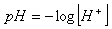pH

The pH scale measures whether a solution is acidic or basic. The pH scale ranges from 0 to 14. A pH of 7 is neutral, less than 7 is acidic, and greater than 7 is basic.

The pH scale is logarithmic and as a result, each whole pH value below 7 is ten times more acidic than the next higher value. For example, a pH of 4 is ten times more acidic than a pH of 5 and 100 times (10 times 10) more acidic than a pH of 6. The same holds true for pH values above 7, each of which is ten times more basic than the next lower whole value. For example, a pH of 10 is ten times more basic than a pH of 9 and 100 times more basic than a pH of 8. pH is determined by the formula